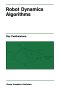# Physics - Impulse

Impulse is the integral of force over time, it is measured in Newton-seconds. For instance a force of one Newton applied over one second will change the momentum, a force of two Newton's applied over half of a second will have a similar effect.

For rigid body collisions we take this to its limit and apply an infinite force over an infinitesimally small time. This impulse is equal to the change in momentum of the colliding objects. Because we are talking about forces here, Newton's third law applies, and the impulse on the colliding objects will be equal and opposite.

impulse = m(vf- vi)

 where: other definitions symbol description type units J impulse vector kg m/s m mass scalar kg vf final velocity vector m/s vi initial velocity vector m/s

This is the intergral of the inertia equation, F=ma

## Impulse and Newtons laws

Impulses have similar laws to forces (as they are time-intergral of force) so they happen in pairs (every impulse has an equal and opposite impulse) and they will be distributed through a framework in a similar way to forces.. Also any net impulse on an object will be ballanced by an impulse on the centre of mass of the object caused by inertia.

As an example, consider a snooker ball being hit off centre by a cue:## Calculating motion from impulse

Assume that we know the value of the impulse and we want to calculate the change in velocity. Note that when we know the initial velocity of the objects we may not know the impulse as described here.

We want to calculate the motion of the rigid body after the impulse as the sum of the linear motion of its centre of mass and its rotation about its centre of mass.

### Linear motion

I think its linear motion is simple, since F = ma = m dv/dt this gives:

Change in velocity = dv = (F/m) dt = impulse/m

So the linear motion will be in the same direction as the impulse, even if the impulse is applied at a point offset from the centre of mass, this seems counter intuitive and this is what I have been trying to get to grips with in the various examples on the website. I think the is correct however, I think that this does not correspond to our intuition because in a practical collision the impulse is not necessarily in the direction of the impact but will have a component which is normal to the surface of the colliding objects.

### Rotation motion

I think we could have an equivalent to Newtons 3rd law for impulses, in other words: every impulse has an equal and opposite impulse. If we apply the impulse to an object which is not attached to anything else then I think this equal and opposite reaction would act on the centre of mass.

Perhaps we could have an angular equivalent of impulse as follows:

Linear impulse = integral(Force) dt = change of linear momentum

Angular impulse = integral(Torque) dt = change of angular momentum

However I think we have to be careful here because in the linear case:

F=ma where m=mass which is a scalar quantity

but in the angular case we have:

T = [I] angAcc where [I] = inertia tensor which is a 3x3 matrix in global coordinates (which will change as the object rotates)

So is it valid to say that:

Change in angular velocity = [I]-1 angular impulse

 where: other definitions symbol description type units [I]-1 the inverse of the inertia tensor in global coordinates (which will change as the object rotates) matrix angular impulse Linear impulse × d bivector d distance between c of m and point where impulse is applied vector m × vector cross product Linear impulse vector

## 6 x 6 Matrix representation

So, in the same way that we did for the inertia equation, we can represent the 3D case

 impRotx impRoty impRotz impx impy impz
=
 ixx ixy ixz 0 -hz hy iyx iyy iyz hz 0 -hx izx izy izz -hy hx 0 0 hz -hy m 0 0 -hz 0 hx 0 m 0 hy -hx 0 0 0 m
 wxf-wxi wyf-wyi wzf-wzi vxf-vxi vyf-vyi vzf-vzi
 where: other definitions symbol description type units ixx ... izz inerta moment components wxf,wyf,wzf final rotation velocity components bivector wxi,wyi,wzi initial rotation velocity components bivector vxf,vyf,vzf final linear velocity components vector vxi,vyi,vzi initial linear velocity components vector m mass scalar hx, hy, hz sx*m, sy*m, sz*m impx, impy, impz linear impulse impRotx, impRoty, impRotz rotational impulse

 metadata block see also: Correspondence about this page Book Shop - Further reading. Where I can, I have put links to Amazon for books that are relevant to the subject, click on the appropriate country flag to get more details of the book or to buy it from them.Robot Dynamics Algorithms (Kluwer International Series in Engineering and Computer Science, 22) Commercial Software Shop Where I can, I have put links to Amazon for commercial software, not directly related to the software project, but related to the subject being discussed, click on the appropriate country flag to get more details of the software or to buy it from them.

This site may have errors. Don't use for critical systems.